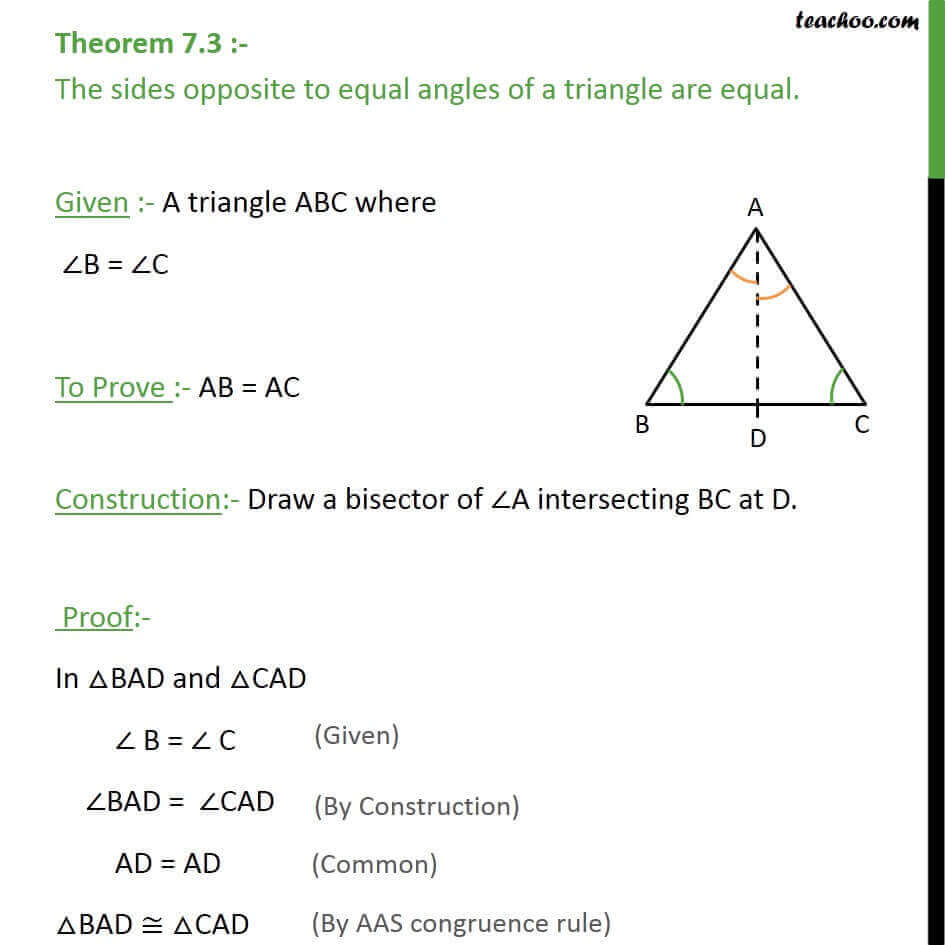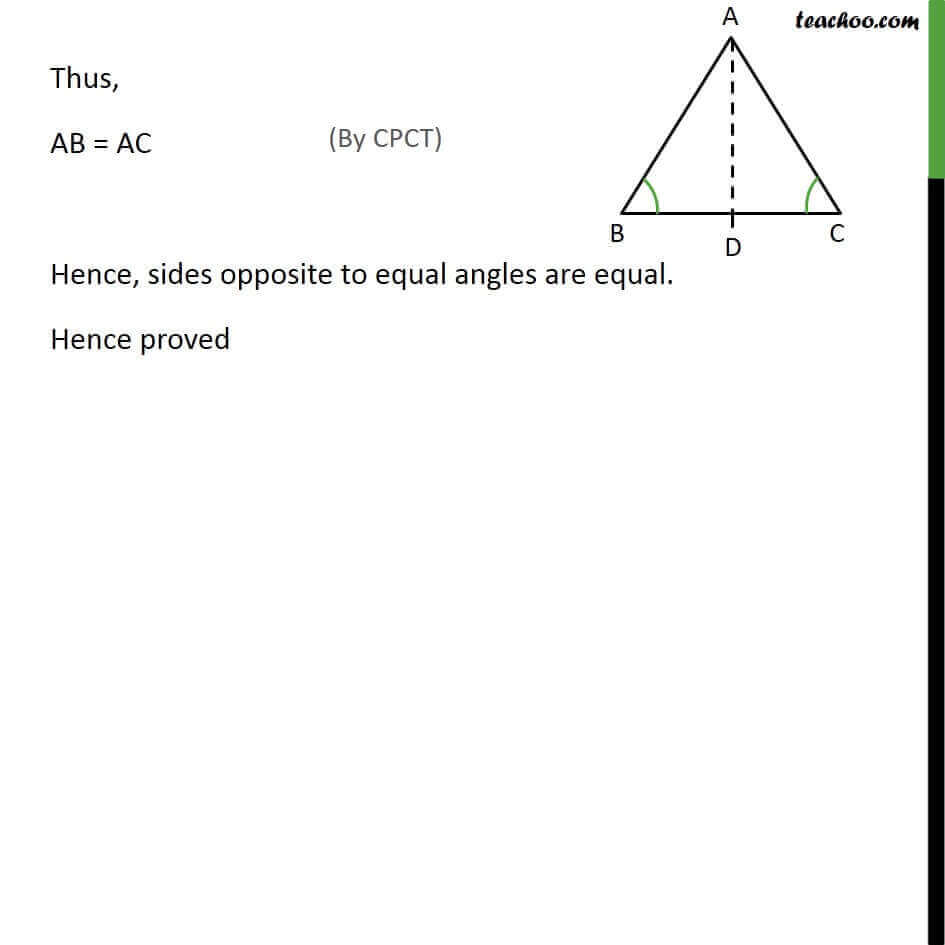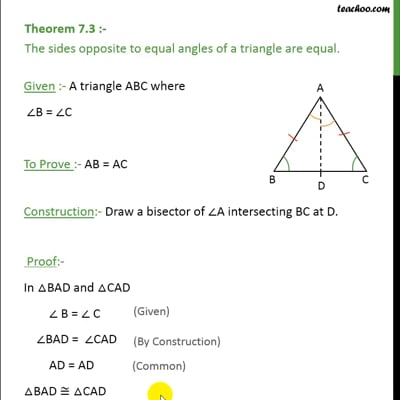Theorems

Chapter 7 Class 9 Triangles
Serial order wiseThis video is only available for Teachoo black users

Learn in your speed, with individual attention - Teachoo Maths 1-on-1 Class

### Transcript

Theorem 7.3 :- The sides opposite to equal angles of a triangle are equal. Given :- A triangle ABC where ∠B = ∠C To Prove :- AB = AC Construction:- Draw a bisector of ∠A intersecting BC at D. Proof:- In △BAD and △CAD ∠ B = ∠ C ∠BAD = ∠CAD AD = AD △BAD ≅ △CAD Thus, AB = AC Hence, sides opposite to equal angles are equal. Hence proved